How Cheenta works to ensure student success?
Explore the Back-Story

# NMTC 2015 Stage II - KAPREKAR (Class 7, 8) - Problems and Solutions###### Problem 1

The diagram above contains 13 boxes. The numbers in the second and the twelfth boxes are respectively 175 and 70 . Fill up the boxes with natural numbers such that

(i) sum of all numbers in all the 13 boxes is 2015 ,
(ii) sum of the numbers in any three consecutive boxes is always the same.
The solution must contain the steps how you arrive at the numbers.
b) if (x, y, z) are real and unequal numbers, prove that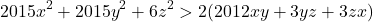###### Problem 2

If a,b,c are reals such that a+b=4 and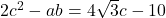, find the numerical values of a, b and c.

###### Problem 3

Whenand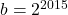, prove that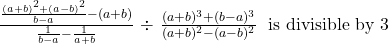###### Problem 4

Prove that the feet of the perpendiculars drawn from the vertices of a parallelogram on to its diagonals are the vertices of a parallelogram.

###### Problem 5

ABC is an acute angled triangle. P,Q are the points onand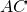respectively such that area of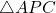= area of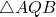. A line is drawn through B parallel to AC and meets the line troughparallel toat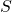.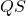cuts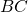at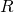. Prove that.

###### Problem 6

(a) A man is walking from a town A to another town B at a speed of. He started one hour before a bus starts. The bus is travelling with a speed of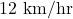. The man on the way got into the bus and travels 2 hours and reached town B. What is the distance between town A and town B.
(b) A point P is taken within a rhombus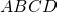such that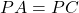. Show thatare collinear.

###### Problem 7

If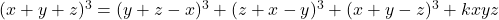find the numerical value of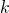. Deduce the following result.

If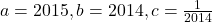prove that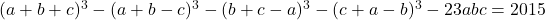###### Problem 1

The diagram above contains 13 boxes. The numbers in the second and the twelfth boxes are respectively 175 and 70 . Fill up the boxes with natural numbers such that

(i) sum of all numbers in all the 13 boxes is 2015 ,
(ii) sum of the numbers in any three consecutive boxes is always the same.
The solution must contain the steps how you arrive at the numbers.
b) if (x, y, z) are real and unequal numbers, prove that###### Problem 2

If a,b,c are reals such that a+b=4 and, find the numerical values of a, b and c.

###### Problem 3

Whenand, prove that###### Problem 4

Prove that the feet of the perpendiculars drawn from the vertices of a parallelogram on to its diagonals are the vertices of a parallelogram.

###### Problem 5

ABC is an acute angled triangle. P,Q are the points onandrespectively such that area of= area of. A line is drawn through B parallel to AC and meets the line troughparallel toat.cutsat. Prove that.

###### Problem 6

(a) A man is walking from a town A to another town B at a speed of. He started one hour before a bus starts. The bus is travelling with a speed of. The man on the way got into the bus and travels 2 hours and reached town B. What is the distance between town A and town B.
(b) A point P is taken within a rhombussuch that. Show thatare collinear.

###### Problem 7

Iffind the numerical value of. Deduce the following result.

Ifprove thatThis site uses Akismet to reduce spam. Learn how your comment data is processed.

### Knowledge Partner